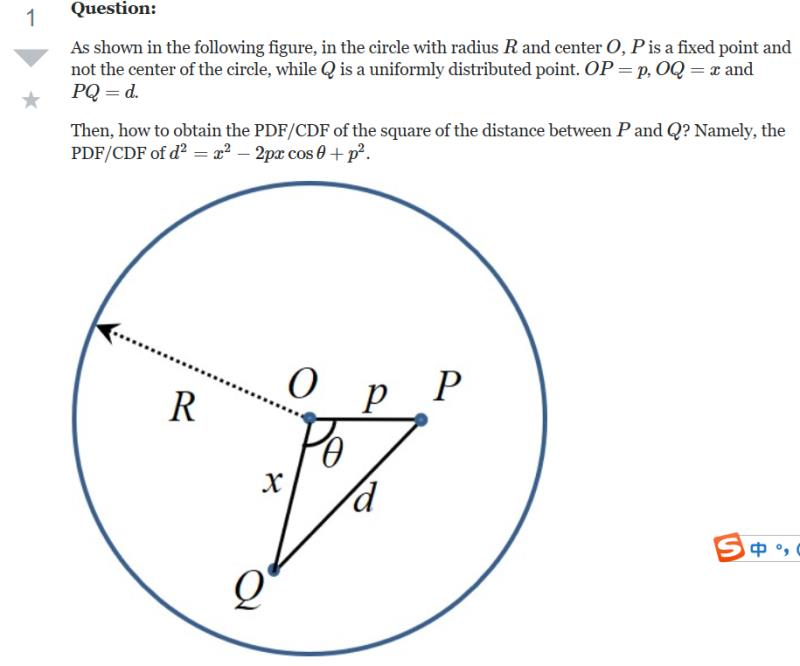Dear Uncle Colin

I have the set-up below - how do I find the cumulative density function for $d^2$?- Circles Don’t Fit

Hi, CDF, and thanks for your message!

Before I start, I’m going to make two simplifying assumptions that don’t change anything ((which is what we mean when we say “without loss of generality”, or “wlog”)) - that the radius of the outer circle $R=1$, and that point P lies on the positive $x$-axis.

This problem breaks down nicely if you think about the CDF as the probability a point in the outer circle lies inside a circle centred on P with a given radius, $d_0$. We’ll deal with the squariness shortly.

There are three ways the circles could be arranged:

• The P-circle could be completely inside the O-circle, if $d_0 \le 1-p$.
• The P-circle could intersect the O-circle, if $1-p < d_0 < 1+p$
• The P-circle could be completely outside the O-circle, if $d_0 \ge 1-p$.

### Case 1: $d_0 \le (1-p)$

If the P circle is inside the O circle, the probability of Q lying inside the P-circle is the ratio of the circles’ areas: $P(d < d_0) = d_0^2$.

### Case 3: $d_0 \ge (1+p)$

If the P circle completely encloses the O circle, Q must lie inside it, so $P(d < d_0) = 1$.

### Case 2: $(1-p) \lt d_0 \lt (1+p)$

This is the interesting situation. To work this out, this diagram is helpful: it shows how the (upper halves of the) two circles interact. In particular, the probability we want corresponds to the area in the intersection of the two semicircles - the triangle and the two regions immediately adjacent to it.

The area of these turns out to be the union of two sectors - one centred on O, with radius 1, and one centred on P, with radius $d_0$. The intersection of the two is the marked triangle - which tells us how to find the area!

We can use cosine rule to determine that:

• $\cos(\theta) = \frac{1+p^2-d_0^2}{2p}$
• $\cos(\phi) = \frac{p^2 + d_0^2 - 1}{2dp}$

The two sectors have areas:

• $A_o = \frac{1}{2}\theta$
• $A_p = \frac{1}{2}d_0^2 \phi$

And the triangle has area $\frac{1}{2}p \sin(\theta)$

Putting those together gives the area of the region as $\frac{1}{2}\left(\theta + d^2 \phi - p\sin(\theta) \right)$.

However, probability-wise, we want the relative area of this compared to the O semicircle, so we need to divide by $\piby 2$.

That gives $P(d < d_0) = \frac{1}{\pi}\left( \theta + d_0^2 \phi - p\sin(\theta)\right)$.

### But what about the CDF of $d^2$?

We know the probability $P(d < d_0) = f(d_0)$ for any value of $d_0$. We want $P(d^2 < k)$ for any value of $k$, and since $d\ge 0$, that’s the same as $P(d < \sqrt{k})$.

In other words, $P(d^2 < k) = f(\sqrt{k})$.

Since $f(d_0) = \dots$

• $\dots d_0^2$ for $0 < d_0 < 1-p$,
• $\dots \frac{1}{\pi} \left( \theta + d_0^2 \phi - p\sin(\theta)\right)$ for $1-p < d_0 < 1+p$
• $\dots 1$ for $1+p < d_0$

… we have $P(d^2 < k) = \dots$

• $\dots k$ for $0 < k < (1-p)^2$
• $\dots \frac{1}{\pi} \left( \theta + k \phi - p \sin(\theta)\right)$ for $(1-p)^2 < k < (1+p)^2$
• $\dots 1$ for $(1+p)^2 < k$

… where $\theta = \cos^{-1}\left(\frac{ 1 + p^2 -k }{2p}\right)$ and $\phi=\cos^{-1}\left(\frac{k + p^2 - 1}{2p\sqrt{k}}\right)$.

At least, that’s what I think I get. Frankly, my eyes have gone wibbly.

Hope that helps!

- Uncle Colin